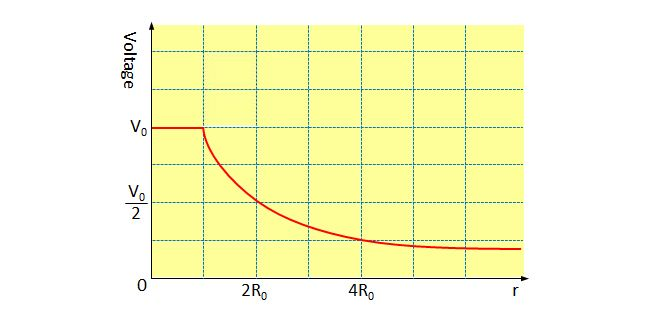# Charged sphereThe above graph shows the electric potential inside or around a charged sphere as a function of $r$ which denotes the distance from the center of the sphere. If the radius of the sphere is $R_0,$ which of the following statements are correct?

a) The charged sphere is charged positively.
b) The intensity of the electric field inside the charged sphere is zero.
c) The intensity of the electric field at $r=2R_0$ is twice as large as that at $r=4R_0.$

×